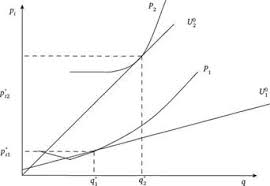## How to Calculate and Solve for Linear Flow Rate in Reservoir Fluid Flow | The Calculator EncyclopediaThe image above represents the linear flow rate.

To compute for the linear flow rate, four parameters are needed and these parameters are Initial pressure (P1), Final Pressure (P2), Thickness (h) and Permeability (k).

The formula for calculating linear flow rate:

q = 0.001127kh[P1 – P2]

Where:
q = Linear Flow Rate
P1 = Initial Pressure
P2 = Final Pressure
h = Thickness
k = Permeability

Let’s solve an example;
Find the linear flow rate when the initial pressure is 12, final pressure is 22, thickness is 18 and permeability is 44.

This implies that;
P1 = Initial Pressure = 12
P2 = Final Pressure = 22
h = Thickness = 18
k = Permeability = 44

q = 0.001127kh [P1 – P2]
q = 0.001127 x 44 x 18 [12 – 22]
q = 0.001127 x 44 x 18 [-10]
q = 0.892584 [-10]
q = -8.92584

Therefore, the linear flow rate is -8.92584 bbl/day.

Calculating Permeability when the linear flow rate, Initial Pressure, Final Pressure and Thickness is Given.

k = q / 0.001127h (p1 – p2)

Where;
k = Permeability
q = Linear Flow Rate
P1 = Initial Pressure
P2 = Final Pressure
h = Thickness

Let’s solve an example;
Find the permeability when the initial pressure is 34, final pressure is 24, thickness is 12 and linear flow rate is 50.

This implies that;
q = Linear Flow Rate = 50
P1 = Initial Pressure = 34
P2 = Final Pressure = 24
h = Thickness = 12

k = q / 0.001127h (p1 – p2)
k = 50 / 0.001127h (34 – 24)
k = 50 / 0.001127h (10)
k = 50 / 0.01127
k = 4436.5

Therefore, the permeability is 4436.5.